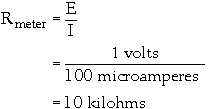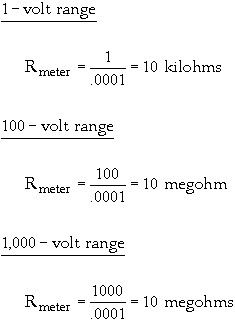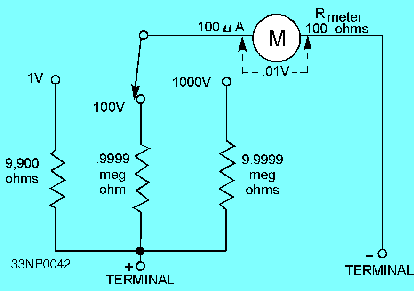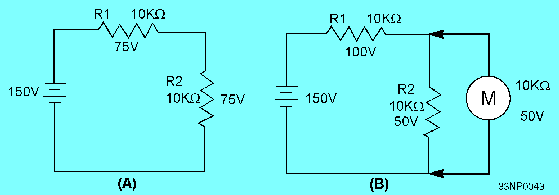basic meter is to be made into a voltmeter with a full-scale deflection of 1 volt.">Custom SearchKeep in mind that the D'Arsonval meter movement uses current flow to produce a magnetic field that is proportional to the current. The meter movement is, therefore, an indicator of current flow rather than voltage. The addition of the series resistance is what allows the meter to be calibrated in terms of voltage; that is, the meter movement of a voltmeter operates because of the current flow through the meter, but the scale is marked in volts. For example, the meter movement shown in figure 3-9 has an internal resistance of 100 ohms, requires 100 microamperes for full-scale deflection, and has a voltage drop of 10 millivolts when full-scale deflection is reached. If you were to place this meter directly across a 10-volt source, an excessive current (in milliamperes) would flow. The meter would be destroyed because of the excessive current flowing through the meter movement. This can be seen in the following Ohm's law application: Figure 3-9. - Use of multiplier resistors with D'Arsonval meter movement.Using this equation, you can see that a current through the meter of 100 milliamperes is excessive and will cause damage.Since the normal voltage drop for the meter is 10 millivolts at full-scale deflection, some means must be supplied to drop the extra 9.99 volts without applying it directly to the meter. This is done by the addition of a multiplier resistor, as shown in figure 3-9. Extending Voltmeter Ranges The value of series resistance is determined by the current required for full-scale deflection and by the range of the voltages to be measured. Since the current through the meter circuit is directly proportional to the applied voltage, the meter scale can be calibrated directly in volts for a fixed value of series resistance. For example, let's assume that the basic meter is to be made into a voltmeter with a full-scale deflection of 1 volt. The coil resistance of the basic meter is 100 ohms, and 100 microamperes of current causes full-scale deflection. The resistance (R meter) required to limit the total current in the circuit to 100 microamperes can be found as follows:Because the meter coil already measures 100 ohms, the series resistance required is equal to 10 kilohms minus 100 ohms, or 9.9 kilohms. Q.16 What factors determine the value of the multiplier resistor?Multirange voltmeters use one meter movement. The required resistances are connected in series with the meter by a switching arrangement. A schematic diagram of a multirange voltmeter with three ranges is shown in figure 3-10 . The total meter resistance (R meter) for each of the three ranges, beginning with the 1-volt range, is figured by the application of Ohm's law, as follows:Figure 3-10. - Multirange voltmeter.The actual value of the multiplying series resistor (Rseries) for each of these circuits is 100 ohms less than the total resistance. This allows for the resistance of the meter coil (Rcoil). Voltmeter Circuit Connections When voltmeters are used, a primary rule of safety is that such voltage-measuring instruments must always be connected in parallel with (across) a circuit. If you are unsure of the level of the voltage to be measured, take a reading at the highest range of the voltmeter and progressively (step by step) lower the range until a suitable reading is obtained. In many cases, the voltmeter you will be using will not be a center-zero- (0 reading is in the center) indicating instrument. Observing the correct polarity is important when connecting the instrument to the circuit. Voltmeter polarity is the same as for the dc ammeter; that is, current flows from negative to positive. Q.17 In what manner are voltage-measuring instruments connected to the circuit to be measured?Influence of a Voltmeter in a Circuit The purpose of a voltmeter is to indicate the potential difference between two points in a circuit. When a voltmeter is connected across a circuit, it shunts the circuit. If the voltmeter has a low resistance, it will draw a substantial amount of current. This action lowers the effective resistance of the circuit and changes the voltage reading. When you are making voltage measurements in high-resistance circuits, use a HIGH-RESISTANCE VOLTMETER to prevent the shunting action of the voltmeter. The effect is less noticeable in low-resistance circuits because the shunting effect is less. The problem of voltmeter shunting (sometimes called circuit loading) is illustrated in figure 3-11. Figure 3-11. - Shunting action caused by a voltmeter.Q.18 When making voltage measurements in a high-resistance circuit, you should always use a voltmeter with what relative value of resistance?Integrated Publishing, Inc. - A (SDVOSB) Service Disabled Veteran Owned Small Business